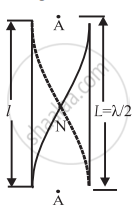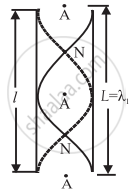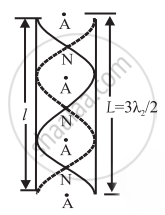# With a neat labelled diagram, show that all harmonics are present in an air column contained in a pipe open at both the ends. Define end correction. - Physics

With a neat labelled diagram, show that all harmonics are present in an air column contained in a pipe open at both the ends. Define end correction.

#### Solution

Consider 'l' length cylindrical pipe that is open at both ends. In a cylindrical open pipe, sound waves are sent down the air column and reflected at the open ends without changing phase. Under the right circumstances, interference between incident and reflected waves creates stationary waves in the air column.

Stationary waves are produced due to the superposition of two identical simple harmonic progressive waves traveling through the same part of the medium in opposite direction. In the case of pipe open at both ends, the air molecules near the open end are free to vibrate, hence they vibrate with maximum amplitude. Therefore each open end becomes an antinode.

Taking into account the end correction e at each of the open ends, the resonating length of the air column is L = I + 2e.

Let v be the speed of sound in air. The simplest mode of vibration is the fundamental mode of vibration. The fundamental tone or mode of vibrations of the air column open at both ends is as shown below figure. There are two antinodes at two open ends and one node between them.The distance between two consecutive antinodes is λ/2, where λ is the wavelength of sound. The corresponding wavelength λ and the fundamental frequency n are

λ = 2L and n = v/λ = v/(2L) = v/(2(l + 2e))     ...(1)

This is the fundamental frequency or the first harmonic. It is the lowest frequency of vibration.

The next possible mode of vibrations of the air column open at both ends is as shown in the below figure. In this case, two nodes and three antinodes are formed. If λ1 and n1 be the corresponding wavelength and frequency,λ= L and n1 = v/(λ_1) = v/(L) = v/(l + 2e) = 2n     ...(2)

This is the frequency of the second harmonic or first overtone.

In the next of vibrations of the air column open at both ends as shown in the below figure, In this case, three nodes & four antinodes are formed. If λ2 and n2 be the corresponding wavelength & frequencyλ2 = (2L)/3 "and"  n_2 = v/(λ_2) = (3v)/(2L) = (3v)/(2(l + 2e)) = 3n  ...(3)

This is the frequency of third harmonic or second overtone.
Therefore, in general, the frequency of the pth overtone (p = l, 2, 3, ... ) is nP = (p + l)n ...(4)

i.e., the pth overtone is the (p + l)th harmonic.

Equations (1), (2) and (3) show that allowed frequencies in an air column in a pipe open at both ends are n, 2n, 3n,... Thus all harmonics are present as overtones in the modes of vibration of air column open at both ends.

Concept: Study of Vibrations of Air Columns
Is there an error in this question or solution?
Chapter 6: Superposition of Waves - Exercises [Page 156]

#### APPEARS IN

Balbharati Physics 12th Standard HSC Maharashtra State Board
Chapter 6 Superposition of Waves
Exercises | Q 9 | Page 156
Share